# CFD Simulations

## Introduction

The TU Darmstadt engine has been investigated by three different groups using LES (Large Eddy Simulation) and hybrid URANS (unsteady Reynolds-averaged Navier-Stokes)/LES. These research groups are located at Technische Universität Bergakademie Freiberg (TUBF), Universität Duisburg-Essen (UDE) and Technische Universität Darmstadt (TUD). In the follow, the different approaches will be presented, including general information about the code and the physical modelling, computational domain and mesh treatment, initial and boundary conditions and computational requirements. Additional and detailed information can be found in the scientific papers listed in Table 3.

## Overview of simulation

Test data for the motored case is available at three different operating points. A test case considering an engine speed of 800 RPM is investigated in the numerical studies, which are summarized in Table 3.

Table 3: Summary of simulations carried out at 800 RPM
Group Approach CFD Code / Publication
TUBF URANS / LES Ansys CFX R16.0 / Buhl et al. [9, 10]
UDE LES OpenFoam-2.3.x / Janas et al. 
TUD LES KIVA-4mpi / He et al. 

Further, simulations were carried out by Nguyen et al.  and Baumann et al. , which are not part of this comparison.

## CFD codes

In the following, the governing equations, turbulence modelling and solver are described for the three different CFD codes.

### Ansys CFX R16.0

For this compressible case, the filtered governing equations for mass, momentum and total enthalpy ${(H=h(T,p)+0.5u_{j}^{2})}$are solved. They are defined as:

 ${{\dfrac {\partial {\overline {\rho }}}{\partial t}}+{\dfrac {\partial \left({\overline {\rho }}{\widetilde {u}}_{j}\right)}{\partial x_{j}}}=0}$${\text{(4.1)}}$${{\dfrac {\partial \left({\overline {\rho }}{\widetilde {u}}_{i}\right)}{\partial t}}+{\dfrac {\partial \left({\overline {\rho }}{\widetilde {u}}_{i}{\widetilde {u}}_{j}\right)}{\partial x_{j}}}=-{\dfrac {\partial {\overline {p}}}{\partial x_{i}}}+{\dfrac {\partial }{\partial x_{j}}}\left(\left(\mu +\mu _{t}\right){\dfrac {\partial {\widetilde {u}}_{i}}{\partial x_{j}}}\right)}$${\text{(4.2)}}$${{\dfrac {\partial \left({\overline {\rho }}{\widetilde {H}}\right)}{\partial t}}-{\dfrac {\partial {\overline {p}}}{\partial t}}+{\dfrac {\partial \left({\overline {\rho }}{\widetilde {u}}_{j}{\widetilde {H}}\right)}{\partial x_{j}}}={\dfrac {\partial }{\partial x_{j}}}\left(\lambda {\dfrac {\partial {\widetilde {T}}}{\partial x_{j}}}+{\dfrac {\mu _{t}}{Pr_{t}}}{\dfrac {\partial {\widetilde {h}}}{\partial x_{j}}}\right)}$${\text{(4.3)}}$with the thermal conductivity ${\lambda }$and the turbulent Prandtl number ${Pr_{t}}$(set to 0.9 in the following). A non-density-weighted filtered variable is denoted by ${\overline {\Phi }}$, while ${\widetilde {\Phi }}$is the density-weighted filtered variable. This system is complemented by the filtered equation of state for perfect gases, defined as:

 ${{\overline {p}}={\overline {\rho }}{\dfrac {R}{W}}{\widetilde {T}}}$${\text{(4.4)}}$with the molecular weight ${W}$and the universal gas constant ${R}$. The eddy viscosity (${\mu _{t}}$) is calculated based on the scale-adaptive simulation (SAS-SST) turbulence model . In case of insufficient spatial or temporal resolution (for a SRS), it reverts to the SST model  and maintains a valid base of modelling. Its key element is the von Kármàn length scale . (${L_{vK}}$), introduced into the scale-determining ${\omega }$equation. In unstable flows, ${L_{vK}}$adjusts the eddy viscosity to a level which allows the formation of a turbulent spectrum . ${L_{vK}}$is defined as

 ${L_{vK}=\kappa {\dfrac {\sqrt {2{\widetilde {S}}_{ij}{\widetilde {S}}_{ij}}}{{\widetilde {u}}''}}}$${\text{(4.5)}}$with

 ${{\widetilde {S}}_{ij}={\dfrac {1}{2}}\left({\dfrac {\partial {\widetilde {u}}_{i}}{\partial x_{j}}}+{\dfrac {\partial {\widetilde {u}}_{j}}{\partial x_{i}}}\right){\text{,}}\quad {\widetilde {u}}''={\sqrt {{\dfrac {\partial ^{2}{\widetilde {u}}_{i}}{\partial x_{k}^{2}}}{\dfrac {\partial ^{2}{\widetilde {u}}_{i}}{\partial x_{j}^{2}}}}}}$${\text{(4.6)}}$and the von Kármán constant ${\kappa }$.

ANSYS CFX R16.0 is a node-based, conservative and time-implicit finite-volume code . The mass flow is evaluated such that a pressure-velocity coupling is achieved . The discrete systems of equations are solved using a coupled algebraic multi-grid method . To minimize numerical diffusion, a second-order scheme in space  and a second-order backward scheme in time are used.

### OpenFOAM-2.3.x

The following governing equations for mass, momentum and absolute internal energy are solved on a moving grid:

 ${{\dfrac {\partial {\overline {\rho }}}{\partial t}}+{\dfrac {\partial \left({\overline {\rho }}{\widetilde {u}}_{j}\right)}{\partial x_{j}}}=0}$${\text{(4.7)}}$${{\dfrac {\partial \left({\overline {\rho }}{\widetilde {u}}_{i}\right)}{\partial t}}+{\dfrac {\partial \left({\overline {\rho }}{\widetilde {u}}_{i}{\widetilde {u}}_{j}\right)}{\partial x_{j}}}={\dfrac {\partial {\overline {\tau }}_{ij}}{\partial x_{j}}}+{\dfrac {\partial \tau _{ij}^{sgs}}{\partial x_{j}}}-{\dfrac {\partial {\overline {p}}}{\partial x_{i}}}}$${\text{(4.8)}}$${{\dfrac {\partial \left({\overline {\rho }}{\widetilde {e}}\right)}{\partial t}}+{\dfrac {\partial \left({\overline {\rho }}{\widetilde {u}}_{j}{\widetilde {e}}\right)}{\partial x_{j}}}+{\dfrac {\partial \left({\overline {\rho }}{\widetilde {K}}\right)}{\partial t}}+{\dfrac {\partial \left({\overline {\rho }}{\widetilde {K}}{\widetilde {u}}_{j}\right)}{\partial x_{j}}}={\dfrac {\partial }{\partial x_{j}}}\left(\alpha _{eff}{\dfrac {\partial {\widetilde {e}}}{\partial x_{j}}}\right)-{\dfrac {\partial }{\partial x_{j}}}\left({\overline {p}}{\widetilde {u}}_{j}\right)}$${\text{(4.9)}}$Here, the overline denotes LES filtered and the tilde Favre filtered quantities. Further, ${{\overline {\tau }}_{ij}}$denotes the viscous stress tensor. Detailed information regarding equations 4.7–4.9 and the utilized variables can be found in .

The standard Smagorinsky model  with a model constant of 0.062 is used to account for the unresolved subgrid stresses τsgs and the Sutherland law is used to calculate the molecular viscosity. The pressure-velocity-density coupling for flows at arbitrary Mach-number, proposed by Demirdžić, is employed to calculate the pressure . Since the time step size is not limited according to the speed of sound, this approach allows bigger time steps, using an implicit second-order scheme. The momentum equation is solved using a central differencing scheme (CDS). In regions with Mach-numbers greater than 0.3 a TVD-scheme is used to avoid numerical problems  and unacceptable numerical dissipation. A total variation diminishing scheme (TVD-scheme) is utilized to discretize the convective scalar-fluxes, using the Sweby limiter .

The time step size employed is calculated from the maximum CFL number of 2. The smallest time step occurs when the valves are opening and closing, since the smallest mesh resolution can be found inside the valve gap and the highest velocities. This leads to time steps smaller than one micro second. After the valves are closed, the time step size increases to almost 50 micro seconds during the compression and expansion stroke.

### KIVA-4mpi

The Favre-filtered governing equations for mass, momentum and internal energy are discretized on a moving mesh according to the Arbitrary Lagrangian-Eulerian (ALE) approach . The overline characterizes LES filtered and the tilde Favre filtered quantities. Further information regarding the different terms of equations 4.10–4.13 can be found in .

 ${{\dfrac {\partial {\overline {\rho }}}{\partial t}}+{\dfrac {\partial \left({\overline {\rho }}{\widetilde {u}}_{j}\right)}{\partial x_{j}}}=0}$${\text{(4.10)}}$${{\dfrac {\partial \left({\overline {\rho }}{\widetilde {u}}_{i}\right)}{\partial t}}+{\dfrac {\partial \left({\overline {\rho }}{\widetilde {u}}_{i}{\widetilde {u}}_{j}\right)}{\partial x_{j}}}}=-{\dfrac {\partial {\overline {P}}}{\partial x_{i}}}+{\dfrac {\partial }{\partial x_{j}}}\left(\left({\overline {\mu }}+\mu _{t}\right)\left({\dfrac {\partial {\widetilde {u}}_{i}}{\partial x_{j}}}+{\dfrac {\partial {\widetilde {u}}_{j}}{\partial x_{i}}}-{\dfrac {2}{3}}{\dfrac {\partial {\widetilde {u}}_{k}}{\partial x_{k}}}\delta _{ij}\right)\right)+{\overline {\rho }}g_{i}$${\text{(4.11)}}$${{\text{with}}\quad {\overline {P}}={\overline {p}}+{\dfrac {1}{3}}{\overline {\rho }}\tau _{kk}^{sgs}}$${{\dfrac {\partial \left({\overline {\rho }}{\widetilde {e}}\right)}{\partial t}}+{\dfrac {\partial \left({\overline {\rho }}{\widetilde {u}}_{j}{\widetilde {e}}\right)}{\partial x_{j}}}=-{\overline {p}}{\dfrac {\partial {\widetilde {u}}_{j}}{\partial x_{j}}}+{\widetilde {\tau }}_{ij}{\dfrac {\partial {\widetilde {u}}_{i}}{\partial x_{j}}}+{\dfrac {\partial }{\partial x_{j}}}\left({\dfrac {\left({\overline {\mu }}+{\dfrac {c_{v}}{c_{p}}}\mu _{t}\right)c_{p}}{Pr}}{\dfrac {\partial {\widetilde {T}}}{\partial x_{j}}}\right)}$${\text{(4.12)}}$The internal energy ${e}$is defined as follows:

 ${e=h-{\dfrac {p}{\rho }}=e_{0}+\int _{T_{0}}^{T}c_{v}dT-{\dfrac {p}{\rho }}\quad {\text{, with}}\quad h=h_{0}+\int _{T_{0}}^{T}c_{p}dT\quad {\text{and}}\quad c_{v}=\left.{\dfrac {\partial e}{\partial T}}\right|_{v}}$${\text{(4.13)}}$The classical Smagorinsky model  with a Smagorinsky constant set to 0.1 is utilized to model the turbulent kinematic viscosity. The quasi-second-order upwind (QSOU) scheme  is used as the spatial and the first-order implicit Euler as the temporal discretization scheme. A staggered arrangement is employed to store the variables and the time step size is adjusted during the computation according to accuracy requirements and to retain a CFL number smaller than one.

## Computational Domain and Mesh Treatment

### Ansys CFX R16.0

As illustrated in Figure 12, the numerical domain is characterized by temporarily disabled ports for the time periods when the corresponding valves are closed. When the valves reopen, the ports are added to the numerical domain. Due to this disabling and enabling strategy, the flow field of each port has to be reinitialized within each individual operating cycle. In this study the reinitialization is done by one (previously calculated) flow field for each port, to minimize the computational costs.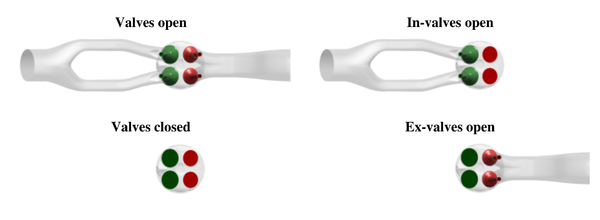Figure 12: Ansys CFX - Numerical domain during the entire operation cycle.

Figure 13 illustrates the mesh topology within the combustion chamber, shown on the valve middle plane. The hybrid, and body-conformal mesh consists of tetrahedral, prism and hexahedral elements with a maximum cell size of 1mm. Mesh refinements of 0.25mm are defined at the intake jet region, the valve stem and the separation edge within the intake port. The boundary layer is resolved by 10 prism layers with a minimum height of 25μm next to the wall. From 310 to 36° bTDC and from 37 to 314° bTDC an extrusion volume is generated to reduce the number of cells. A key grid approach  is used, while the effects of mesh morphing and interpolation were investigated in a previous study . The time step width is set to 0.1 CAD (≈ 2.08×10-5s) for all simulations.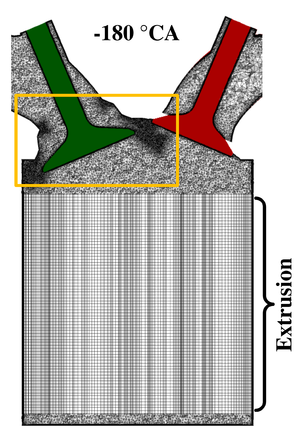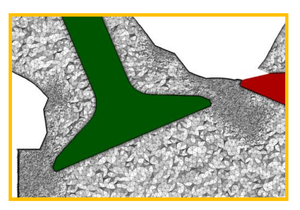Figure 13: Ansys CFX - General mesh topology within the combustion chamber. Mesh refinement at the intake jet region, valve stem and intake port separation edge are highlighted by orange box.

### OpenFOAM-2.3.x

The numerical grid is built up of equidistant hexahedral cells, locally refined at the spark plug and the valve seat region (see left of Figure 14. Inside the combustion chamber an average cell size of 0.5mm is employed, whereas inside the valve seat region, the cell size is 0.125 to 1mm. At the spark plug the cell size is 0.25mm and inside the inlet and outlet manifolds, at a distance of the valves, the mesh resolution is reduced to 2mm. In total, the used grids contain 2.0 million cells at TDC and up to 5.7 million cells at BDC. To close the valves, so called 'curtains' are used, which are internal walls around the valve seat.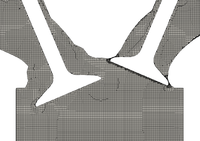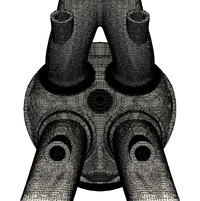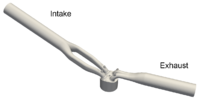Figure 14: OpenFoam - Left: Engine grid on a cut through the valves with opened intake valve. Middle: Top view of the cylinder head. Right: Full computational domain.}

The mesh motion strategy involves 144 target grids for every 5 CAD for one full cycle, which are created prior to the simulation. Here, 5 CAD correspond to a piston motion of less than 4mm, where the mesh quality stays high. Every 5 CAD the results are mapped to a new grid. The mesh motion is realized by shifting each mesh point by a distance resulting from the multiplication of the time step size and deformation velocity, which is obtained by a Laplace equation for a grid velocity field. Further, all convective fluxes are calculated relative to the moving grid . Further Information can be found in .

### KIVA-4mpi

The computational domain involves the complete geometry of the IC engine including intake and exhaust till the measuring locations (in case of intake second measurement location), see Figure 2. The mesh consists of hexahedral elements with a varying resolution within the engine geometry which is displayed in Figure 15. At BDC, the averaged cell width around the intake valves is approximately 0.4mm and 0.72mm in the cylinder body. Due to the mesh movement, the total amount of cells varies between 0.41 million cells at TDC and 1.76 million cells at BDC. Details about the mesh motion strategy can be found in .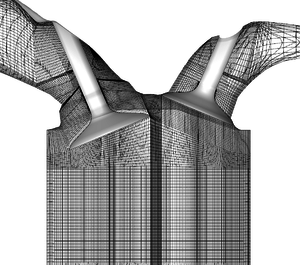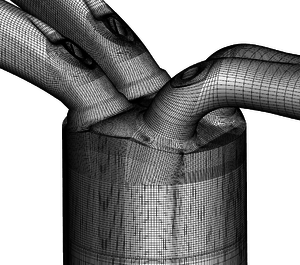Figure 15: KIVA-4mpi - Left: Mesh on valve middle plane (z=19mm) at 270° bTDC.Right: Isometric view of cylinder head.

## Initial and Boundary Conditions

### Ansys CFX R16.0

The boundaries are illustrated in Figure 16. For the exhaust port, time-resolved pressure and temperature curves were chosen. The intake port boundary condition consists of a time-resolved temperature and mass flow. The fluid within the fireland crevice (clearance volume between cylinder and piston) is modelled based on the time-resolved mass flow and temperature at the annular gap derived from the piston clearance (0.53mm), see right of Figure 16. Please note that blow-by gases are neglected in this work. If not available in the experiment, the boundary conditions were generated by previous 0D/1D simulations, which will be described next.

Figure 17 illustrates the general workflow to achieve the boundary conditions and initialization data. First (S1), a 0D/1D engine model up to the pressure plenum was created using the software GT-Power (v7.2). This model was calibrated with the available experimental data (e.g. in-cylinder pressure) and time-resolved boundary conditions were extracted at defined positions (indicated in Figure 17. In a second step (S2), an URANS simulation was performed to obtain the necessary port flow fields. Synthetic turbulence  was used to generate 6 different initial fields for SRS. Afterwards, 3 consecutive cycles were simulated for each of these 6 initial fields. In a last step (S3), the flow fields obtained were used to initialize the consecutive operating cycles.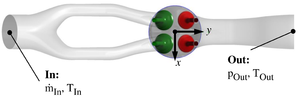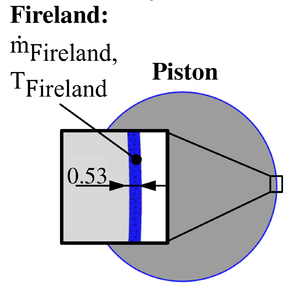Figure 16: Ansys CFX - Boundary condition imposed on the intake and exhaust ports. Right: Blue area on piston indicates the piston clearance volume used for fireland crevice modeling.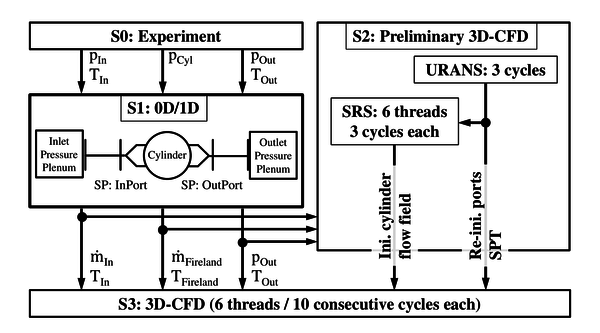Figure 17: Ansys CFX - Workflow to obtain boundary conditions and initialization data.

### OpenFOAM-2.3.x

At 360° bTDC the intake and exhaust valves are open and the fluid is assumed to be at rest in the entire domain. At this point the simulation is started and 20 consecutive engine cycles are calculated. From the measurements, the time-varying absolute pressure is known and imposed at positions of 530mm upstream of the intake valves and 360mm downstream of the exhaust valves. The piston top-land crevice volume was blocked and the geometric compression ratio adapted, accordingly. At the walls a no-slip boundary condition is applied. The temperature is set to 295K at the inlet and to a fixed value of 333K at the walls. No additional wall modelling is used considering the heat transfer.

### KIVA-4mpi

The initial temperature and pressure in the intake and exhaust pipe as well as the time-dependent pressure boundary condition are set to the experimentally determined values at the second intake and exhaust measuring location shown in Figure 2. The piston top-land crevice volume is included in the computational domain wherefore no additional boundary condition is needed here. The valve temperature is set to 338K and the wall temperature of all other walls to 300K. Further, a no-slip condition is applied and no special treatment of the walls is performed.

## Computational Requirements

### Ansys CFX R16.0

The simulations were performed on INTEL E5-2680v2 processors connected by InfiniBand FDR. Table 4 summarizes the computational effort for the simulated 60 cycles. Accordingly, approximately 5.79 days were required for the simulation of one full cycle.

 Minimum number of finite volumes 1.8×106 Maximum number of finite volumes 8.7×106 Average number of finite volumes 4.9\$×106 CPU 120 CPUh 1.0×106

### OpenFOAM-2.3.x

The simulations were performed on 16 CPUs (AMD Opteron - Type 6234) with a total of 192 cores. Table 5 summarizes the computational time required for the different phases of the cycle.

Table 5: OpenFoam - Computational time for one engine cycle presented for the different time intervals: valve overlap, intake valve closing, exhaust valve closing
Interval days % of total time CAD
Full cycle 6.5 100 720
Intake valve closing 1.1 16 30
Exhaust valve opening 2.4 38 45
Overlapping 0.5 7 50
Rest 2.4 37 595

### KIVA-4mpi

In general, the simulation were performed on an Intel Xeon Processor E5-2680 v3 using 12 CPU. A cycle-parallelization technique was used to calculate several runs of consecutive cycles, further described in He et al. . Considering this, approximately 78.23 hours were required to calculate one cycle using the described mesh.

Contributed by: Carl Philip Ding, Rene Honza, Elias Baum, Benjamin Böhm, Andreas Dreizler — Fachgebiet Reaktive Strömungen und Messtechnik (RSM),Technische Universität Darmstadt, Germany

Contributed by: Brian Peterson — School of Engineering, University of Edinburgh, Scotland UK

Contributed by: Chao He, Wibke Leudesdorff, Guido Kuenne, Amsini Sadiki, Johannes Janicka — Fachgebiet Energie und Kraftwerkstechnik (EKT), Technische Universität Darmstadt, Germany

Contributed by: Peter Janas, Andreas Kempf — Institut für Verbrennung und Gasdynamik (IVG), Lehrstuhl für Fluiddynamik, Universität Duisburg-Essen, Germany

Contributed by: Stefan Buhl, Christian Hasse — Fachgebiet Simulation reaktiver Thermo-Fluid Systeme (STFS), Technische Universität Darmstadt, Germany; former: Professur Numerische Thermofluiddynamik (NTFD), Technische Universität Bergakademie Freiberg, Germany